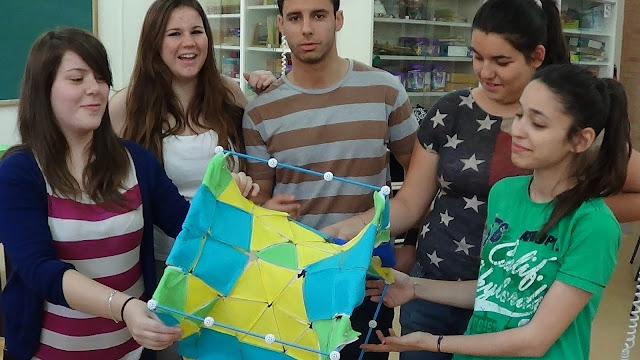Sunday, May 19, 2013

TESSELLATIONS OF SURFACES WITH 3DPOLYFELTScherk surface model with 3D Polyfelt, fixed on a ZOME cube.
As announced in "Covering a float with 3DPolyfelt" we have continued working on tessellations of surfaces with our flexible pieces of felt. There are many figures left, but here are some surfaces.

Session 1: (May 16th, 2013)
We have "covered" several surfaces with regular polygons: cylinders, cones, toruses and some hyperbolic surfaces, like the Scherk surface. It has been a very interesting experience, that we would like to share with other Math teachers of primary and secondary schools. More advanced sessions for high schools or university level can be also done, as we will see below.

Students can recognize immediately different types of curvatures depending on the configuration of polygons around each point. One can see that around a FLAT point, the sum of the angles is exactly 360º. Around a PARABOLIC point, like in any convex polyhedron, the sum has to be <360º. And around a HYPERBOLIC point the sum must be > 360º. The difference from 360º is called defect (or excess) of the vertice (see example below, added on May 22nd).

The next step could be to find all possibe combinations for each of these three types of points. This would lead, for instance, to the classification of semiregular mosaics, or the Archimedian polyhedra. The concepts of Gauss and mean curvatures could be also introduced, if one has soap bubbles, like in our case. [Added on May 22nd: We thank David Crespo tell us his experience with semiregular mosaics at his school.]

Another interesting concept is the Euler characteristic (X= vertices-edges+faces) of  the constructed tessellations. Here the teacher explains that the Euler characteristic of the platonic solids is 2, and other simple examples. In general, one can check if the formulas X=2-2g-r (respectively, X=2-g-r) if the surfaces are orientable (respectively, non orientable), where g is the genus of the surface and r is the number of boundary components.

Session 2: (May 21st, 2913)
We have continued working on the torus, since this was not successfull in the previous session. This time, my students of Differential geometry of Curves and Surfaces, of 3rd year of Mathematics have got tessellations for two floats of different sizes. And you can see the result in this video!

Session 3: (May 22nd, 2013)
As we know, the torus is an orientable surface of genus g=1, without boundary (r=0), hence its Euler characteristic is X=2-2g-r=0. This can also be computed with any tessellation. By a carefully counting, our tessellation of the small float has 27 vertices, 113 edges, and 86 triangles. This gives X= 27-113+86=0.

We can also apply the discrete Gauss-Bonet theorem (a generalization of Descartes's theorem) to get the Euler characteristic of the torus (times 2 Pi), which is 0. For that we have to compute the angular defect / excess of all the vertices. Recall that the angular defect (if possitive, or excess, if negative) of a vertice is equal to 2 Pi minus the sum of the angles of the polygons around.

In our particular tessellation of the small float, we have
• 11 flat vertices, i.e. with 6 (equilateral) triangles around. The defect of such vertices is 2 Pi - 6 (Pi/3) =0, so these do not count.
• 9 parabolic vertices, with 5 triangles around. The excess of each vertice is 2 Pi - 5 (Pi/3)= Pi/3, hence the total defect is 9 Pi/3.
• 7 hyperbolic points, 2 of 8 triangles, and 5 of 7 triangles. Each vertice of 8 triangles gives an  excess of 2 Pi -8 (Pi/3) = -2 Pi/3. Each vertice of 7 triangles, 2 Pi - 7(Pi/3) = - Pi/3. The sum of all excesses is -9 Pi/3.

Hence, the sum of all excesses and defects is 0, as desired.

We have seen two different easy ways to compute the Euler characteristic of a surface.

Excercise: Can you do the same for our tessellation of the big float? You can also try with the Stewart torus.

Participants of these sessions:
Isabel María Romero ( Departamento de Didáctica, de la Universidad de Almería),
David Crespo (del colegio Agave, de Huercal de Almería)
and myself,
Students of the subject "La geometría y la medida en educación primaria", of the Grado en Educación Primaria, of the University of Almería.
Students of high school Agave, Almería
Students of 3th degree of Mathematics.

PD: Esta entrada participa en la Edición 4.1231 del  Carnaval de Matemáticas, cuyo blog anfitrión es i-matematicas.